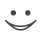# Hol' Dir diese Lernkarten, lerne & bestehe Prüfungen. Kostenlos! Auch auf iPhone/Android!

E-Mail eingeben: und Kartensatz kostenlos importieren.

## Math Flashcards (25 Karten)

n+0=n
Identity Property of Addition
Subtraction Property of Inequality
For every real number a,b, and c, if a>b, then a-c> b-c; if a<b, then a-c< b-c
(a*b)*c = a*(b*c)
Associative Property of Multiplication
Addition Property of Equality
For every real number a, b, and c, if a=b then a+c=b+c
a>b, then a+c>b+c
Addition Property of Inequaltiy
Subtraction Property of Equality
fir every real number a, b, and c, if a=b then a-c=b-c.
n+(-n)=0
Inverse Property of Addition
Multiplication Property of Equality
For every real number a, b,and c, if a=b then a*c=b*c
1*n=n
Identity Property of Multiplication
Multiplication Property of Inequality
For every real number a and b, and for c>0, if a>b then ac>bc; if a<b, then ac<bc.
For every real number a and b, and for c<0, if a>b, then ac<bc; a<b, then ac<bc
n*0=0
Multiplication Property of Zero
Divison Property of Equality
For every real number a, b, and c, with c not equal to 0, if a=b then a/c=b/c
-1*n=-n
Multiplication Property of -1
Divisoin Property of Inequality
For every real number a and b, and for c>0, if a>b, then a/c > b/c; if a<b, then a/c < b/c
For every real number a and b, and for c>0, if a<b, then a/c < b/c; if a>b, then a/c>b/c
a(1/a)=1
Inverse Property of Multiplication
Reflexive Property of Equality
Forevery real Number a, a=a
a(b+c)=ab+ac
Disributive Property
Symmetric Property of Equality
For every real number a and b, if a=b then b=a
a+b = b+a
Commutative Property of Addition
Transitive Property of Equality
For every real number a, b, and c, if a=b and b=c, then a=c
a*b = b*a
Commutative Property of Multiplication
Transitive Property of Inequality
For every real number a, b, and c, if a<b and b<c, then a<c
n+0=n
Identity Property of Addition
Transitive Property of Inequality
For every real number a, b, and c, if a<b and b<c, then a<c
(a+b)+c = a+(b+c)
Associative Property of AdditionKartensatzinfo:
Autor: CoboCards-User
Oberthema: Mathematics
Thema: General
Veröffentlicht: 12.01.2011

Schlagwörter Karten:
Alle Karten (25)
keine SchlagwörterMissbrauch melden